# Structure and dynamics of the molten alkali-chloride salts from an X-ray, simulation, and rate theory perspective†

Santanu Roy*a, Fei Wu b, Haimeng Wangc, Alexander S. Ivanov*a, Shobha Sharma b, Phillip Halstenbergad, Simerjeet K Gill e, A. M. Milinda Abeykoonf, Gihan Kwonf, Mehmet Topsakal e, Bobby Layne g, Kotaro Sasakig, Yong Zhang c, Shannon M. Mahurina, Sheng Daiad, Claudio J. Margulis*b, Edward J. Maginn*c and Vyacheslav S. Bryantsev*a
aChemical Sciences Division, Oak Ridge National Laboratory, 1 Bethel Valley Rd., Oak Ridge, TN 37830, USA. E-mail: roys@ornl.gov; bryantsevv@ornl.gov; ivanova@ornl.gov
bDepartment of Chemistry, The University of Iowa, USA. E-mail: claudio-margulis@uiowa.edu
cDepartment of Chemical and Biomolecular Engineering, University of Notre Dame, USA. E-mail: ed@nd.edu
dDepartment of Chemistry, University of Tennessee, Knoxville, USA
eNuclear Science and Technology Department, Brookhaven National Lab, USA
fNational Synchrotron Light Source II (NSLS-II), Brookhaven National Lab, USA
gChemistry Division, Brookhaven National Lab, USA

Received 9th July 2020 , Accepted 13th August 2020

First published on 14th August 2020

Molten salts are of great interest as alternative solvents, electrolytes, and heat transfer fluids in many emerging technologies. The macroscopic properties of molten salts are ultimately controlled by their structure and ion dynamics at the microscopic level and it is therefore vital to develop an understanding of these at the atomistic scale. Herein, we present high-energy X-ray scattering experiments combined with classical and ab initio molecular dynamics simulations to elucidate structural and dynamical correlations across the family of alkali-chlorides. Computed structure functions and transport properties are in reasonably good agreement with experiments providing confidence in our analysis of microscopic properties based on simulations. For these systems, we also survey different rate theory models of anion exchange dynamics in order to gain a more sophisticated understanding of the short-time correlations that are likely to influence transport properties such as conductivity. The anion exchange process occurs on the picoseconds time scale at 1100 K and the rate increases in the order KCl < NaCl < LiCl, which is in stark contrast to the ion pair dissociation trend in aqueous solutions. Consistent with the trend we observe for conductivity, the cationic size/mass, as well as other factors specific to each type of rate theory, appear to play important roles in the anion exchange rate trend.

## 1 Introduction

Molten salts are resurging as integral components of clean, sustainable, and energy-efficient technologies.1 Their utilization as electrolytes in advanced intermediate- and high-temperature (>200 °C) batteries facilitates attaining high energy density and high power density typically required for electric vehicles and concentrating solar power plants.2 Molten salts can operate as coolants in nuclear reactors at high temperatures (500–900 °C) but near ambient pressure, ensuring efficient and safe operation of the reactors.3,4 Furthermore, different eutectic mixtures of molten salts are promising solvents for electrorefining of nuclear wastes, chemical separation of actinides and rare earths, metal production, alloy heat treatment, and electrochemical synthesis of carbonaceous materials.1,5–8 Enabling this plethora of potential applications requires in-depth understanding of the correlation between microscopic structure, dynamics and macroscopic transport properties such as diffusivity and conductivity.9,10

This article focuses on the alkali-chloride family of molten salts for which we make predictions on what the important driving forces are for transport. The accuracy of these predictions strongly depends on the quality of our simulations. For example, the 2D free energies that will be introduced in our rate theory study are directly related to the structural distributions of ions in different coordination shells. A comparison between computed and measured X-ray/neutron scattering data including structure functions and concomitant pair distribution functions (PDFs)11 can help validate the accuracy of models and simulations in determining molten salt coordination environments and associated free energies.12–17 Early experimental data already exist for monovalent chloride salts obtained through pioneering neutron diffraction experiments employing the isotopic substitution technique, albeit with limited resolution and momentum transfer (q) range.18–23 Modern X-ray diffraction studies on the simple chloride melts are scarce, and old data were typically obtained from standard X-ray sources with a narrow q-range,24,25 causing uncertainties in the positions and heights of peaks in the corresponding PDF due to truncation effects.11 In our current study, we overcome these obstacles by utilizing high-energy X-rays (λ = 0.1667 Å, 74.4 keV) to measure structure functions, S(q), with an extended q-range and low statistical noise, which by Fourier transformation provide more accurate PDFs.

One of the critical objectives of molten salt-based technologies in electrochemical applications is to attain high ionic conductivity.26,27 Trends in diffusivities and conductivities will be contrasted with available experimental data in the literature allowing us not only to test models but also to establish possible connections with rate theories28–54 of ion exchange. Several computational studies on molten alkali halide salts and their mixtures at different concentrations and temperatures already exist in the literature,9,55–70 but questions still remain about transport and ion exchange dynamics on a microscopic scale. For example, it has been established that a small cation (such as Li+) in neat molten salts has high internal mobility, which is reduced significantly when mixed into a salt of a larger cation (such as K+) at a low mole fraction of the smaller cation.63,68,71 Although this finding about the cationic dynamics is remarkable, rates and mechanisms describing the dynamics of anion-exchange around cations remain unknown even in the case of the neat salts. We will attempt to obtain such mechanistic information and reveal the effect of barriers, mass, volume, and the coupling to solvent by applying and in cases extending the formalism of Marcus theory28–36 and transition state theory.37–39,42–45

## 2 Materials and experiments

### 2.1 Molten salt samples

The anhydrous monovalent chloride salts LiCl, NaCl, and KCl (99.9% purity) were purchased from a commercial supplier. Approximately 50 grams of each salt were loaded into quartz tubes in a glove box under a nitrogen atmosphere. Each tube was fit with a compression fitting connected to a Teflon stopcock, taken out of the glove box, and connected to a vacuum line. The valve was opened to allow for the tube containing the salt to be evacuated to a pressure of 1 × 10−3 Torr. When the desired vacuum was reached, each salt was melted and kept at a temperature of 825 °C for ∼15 minutes under dynamic vacuum. The tube was then taken back into the glove box and each salt recovered and stored under a nitrogen atmosphere for further experimentation.

The containment used for the X-ray scattering experiments was a cylindrical quartz capillary of 1 mm internal diameter and 0.01 mm wall thickness. Each capillary was fitted with a quartz tube of 4 mm internal diameter. The addition of the extra length of quartz tubing allowed for the attachment of a compression fitting so that vacuum could be pulled to flame seal the capillaries, once they were loaded with samples (Fig. S1 in the ESI). After the extra tubing was fitted to each capillary, they were taken into the glove box. Each salt was crushed into a fine powder using a mortar and pestle and then the additional tubing added to each capillary was used as a funnel, and the powder was added until the capillary was full. After the capillary was packed with the appropriate amount of salt, it was taken out of the glove box with a compression fitting and flame sealed under a 1 × 10−3 Torr vacuum.

### 2.2 X-ray scattering measurements

Total X-ray scattering experiments were performed at the National Synchrotron Light Source II (NSLS-II), 28-ID-1 beamline, using X-ray photons of wavelength 0.1667 Å and a beam of cross-sectional area 0.25 × 0.25 mm2, giving an accessible q-range up to 28 Å−1. The complete experiment comprised the measurement of the X-ray diffraction patterns for a molten salt in a cylindrical quartz capillary and the empty capillary. Data were collected using a customized furnace (details are reported in our previous work on molten salts69) to hold a quartz capillary at high temperature (958 K (LiCl), 1148 K (NaCl), and 1173 K (KCl)). These temperatures were explicitly selected to compare our new results with previously reported X-ray/neutron scattering experiments.18,20,21,24 Calibration of the precise sample to detector distance (207.68 mm), detector tilt and rotation, and beam center was performed using a Ni powder as the standard. The measured background intensity was subtracted, and standard corrections11,72 were made for inelastic Compton scattering and sample self-attenuation using the PDFgetX2 software.73 The obtained data were subsequently normalized to the average electron density given by the weighted sum of the atomic X-ray form factors, yielding the total structure function S(q):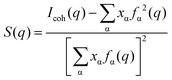(1)
where Icoh is coherent scattering intensity, xα and fα(q) are the molar fraction and q-dependent X-ray atomic form factor of species α, respectively, and q denotes the magnitude of the scattering vector (q = (4πsinθ)/λ, where 2θ is the scattering angle, and λ is the incident X-ray wavelength). Notice that in the current article we define the structure function S(q) as in ref. 69 and 70. As defined here, S(q) goes to zero at large q and corresponds to what in prior literature74 Keen refers to as Fx(q), “the X-ray weighted total-scattering structure factor”.74 In other words, our S(q) is Fx(q), and we provide this remark here to avoid any confusion with different terminologies and definitions commonly used in total scattering.

In this study, the reduced pair-distribution function (D(r)) in the range qmin = 0.6 Å−1 to qmax = 16 Å−1 is defined72 as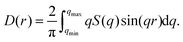(2)
This definition has the operational advantage that direct comparison can be made between simulations and experiments without the need to explicitly measure the number density ρ0, which is already contained in D(r) as the slope of the function at low r.72 Experimental S(q) and D(r) for the entire q-range (up to 18 Å−1) and r-range (up to 20 Å) considered in our experiments are presented in Fig. S2 in the ESI. A subcomponent of D(r) is defined as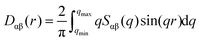(3)
where Sαβ is the partial structure function defined in ref. 69 and 70.

## 3 Simulation protocol

To interpret our X-ray measurements and to better understand the validity of different models, we carried out three different types of MD simulations based on (1) density functional theory (i.e. ab initio MD (AIMD)),75 (2) a non-polarizable ‘rigid’ ion model (RIM),76–79 and a polarizable ion model (PIM).69,80,81 As it will become apparent, structural information from simulations at different levels of theory shows reasonably good agreement with our experimental findings, providing confidence in the interpretation of experimental observations. Due to the prohibitive cost associated of collecting sufficient statistics, transport properties including diffusion coefficients and ionic conductivities were computed using both the PIM and RIM but not AIMD.

### 3.1 MD simulations using the RIM and PIM

To study the structure and dynamics of LiCl, NaCl, and KCl, simulation boxes containing 864 ion pairs were generated. Initial configurations were those of the crystal structures of the salts at the experimental liquid densities. For convenience, all systems were first equilibrated using the RIM.76–79 Crystals were first melted at 3000 K and then cooled to target temperature (958 K for LiCl, 1148 K for NaCl, and 1173 K for KCl) over 5 ns at constant volume. After this, systems were further equilibrated in the constant pressure and temperature (NPT) ensemble for another 3 ns (at 1 atm and target temperature). The last 1 ns of these runs was used to compute S(q) (see ref. 69 for details), as well as D(r) and its subcomponents. RIM simulations were performed using the LAMMPS package82 with a time step of 1 fs.

Final snapshots from the RIM simulations were taken as initial points for simulations using the PIM.80,81 Each system was first subject to a 200 ps simulated annealing equilibration at constant pressure (at 1 bar) that increased their temperature to 1640 K and cooled back to target temperatures. Further equilibration and production runs in the NPT ensemble (at 1 bar and target temperature) as well as the calculation of S(q) and D(r) followed the same scheme as described for the RIM simulations. PIM simulations were performed using the CP2K package83 with a time step of 1 fs. Both the RIM and PIM simulations used the Nosé–Hoover thermostat and barostat84–86 with a time constant of 1 ps to control the temperature and pressure. The non-bonded cutoff was set to 15 Å.

Additionally, following a similar protocol to the one described above, all system were equilibrated to a target temperature of 1100 K. The last 2 ns of 3 ns runs in the NPT ensemble were used to compute average densities. Volumes in the last snapshot of these NPT runs were rescaled to match these average densities and used as starting points for constant volume–temperature (NVT) runs. In the case of the RIM (PIM), we further equilibrated the system for 1 ns (0.5 ns) in the NVT ensemble and collected data from a production run of 1 ns in duration for computing 2D-free energy surfaces. We verified convergence by noting that any 100 ps segment could reproduce identical 2D-free energy surfaces.

### 3.2 AIMD simulations

AIMD simulations are significantly more expensive than those using the RIM and PIM. Hence, the protocol was to run these at the aforementioned target temperatures and corresponding experimental densities (1.469 g cm−3 for LiCl, 1.516 g cm−3 for NaCl, and 1.452 g cm−3 for KCl)87 directly in the NVT ensemble. To provide the best possible initial configuration for these runs, we first equilibrated 149 ion pairs in the NPT ensemble at 1 bar using the PIM. We then selected an equilibrated configuration from the NPT trajectory for which the density coincided with the experimental one and used it as initial structure for subsequent AIMD.

AIMD simulations used the Quickstep module of the CP2K software,75 where trajectories were run in the NVT ensemble using the Nosé–Hoover thermostat with a 1 ps time constant.84,85,88 Valence electrons were treated explicitly at the DFT level employing the revPBE functional89 and the DZVP-MOLOPT basis set90 with density from a cutoff of 400 Ry. The core electrons on all atoms were represented by revPBE pseudopotentials, and we used the Grimme's D3 long-range dispersion correction to the DFT functional.91 A time step of 0.5 fs was used to generate a 120 ps trajectory and the last 100 ps of that trajectory were used for computing S(q) and D(r).

### 3.3 Calculation of transport properties

Diffusion coefficients were calculated from 1 ns RIM and PIM simulations at 1100 K in the NVT ensemble with density selected as the average resulting from NPT equilibration as described in Section 3.1 using the expression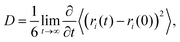(4)
where (ri(t) − ri(0))2 represents the squared displacement of ion i at time t, and the 〈…〉 indicates an ensemble average for all ions of the same ion type. Since the diffusion coefficient is system-size dependent, to get a best estimate to its large size limit we applied the Yeh–Hummer correction92 as defined in eqn (5).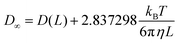(5)
In eqn (5), D is the infinite system size diffusion coefficient, D(L) is the calculated diffusion coefficient from a cubic box with edge length L, and kB, T, η are the Boltzmann constant, temperature and shear viscosity respectively. For the different models, η values used in eqn (5) were computed using a protocol similar to that described in ref. 93.

To compute the ionic conductivity of the RIM and PIM, 30 independent trajectories were simulated using the same NVT simulation protocol and data were saved every 10 fs for analysis. These trajectories were 1 ns in duration in the case of the RIM and 200 ps in the case of the PIM. Data at 100 fs intervals from these 30 independent trajectories were also used to evaluate standard deviation errors in the calculated diffusion coefficients.

Ionic conductivity was computed using the Green–Kubo formula: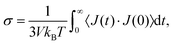(6)
where,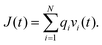(7)
Here, J(t) is the charge flux, qi is the formal charge of each ion, and vi(t) is the velocity of each ion. The ionic conductivities were computed from the average of the 30 independent trajectories. To estimate errors in our conductivity calculations, a bootstrapping method was utilized in which 30 trajectories were randomly selected 50 times, (a given trajectory can be selected multiple times) and the standard deviation was computed from the result of these 50 trials. We also report the computed the molar conductivity as defined in eqn (8).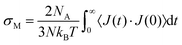(8)
Here, NA and N are the Avogadro number and the total number of ions, respectively.

### 3.4 Rate theories for anion exchange

In an anion exchange process around a cation, the equilibrium coordination structure of the cation before the exchange is the same as its equilibrium coordination structure after the exchange. However, since we want to develop a Marcus-based theory for our systems we need reactants and products with coordination states that are distinct. Such distinction can be artificially introduced by theoretically treating a selected cation–anion pair as the solute and the rest of the ions in the system as part of the solvent. Of course, any neighboring anion can be considered as the selected counter-ion and this is effectively what we do upon averaging. For the purpose of developing our model, we consider a 2D-reaction coordinate Rc = (r,n), involving (1) the cation–anion distance (r) of the selected ion pair and (2) the number of remaining anions (n) from the solvent coordinating with the cation of this pair. For this reaction coordinate, reactant, product and transition state are pictorially depicted in Fig. 1 as concentric, alternatively arranged, anionic and cationic solvation shells around the cation X+. In Fig. 1, the selected Cl (labeled red) that forms a hypothetical solute ion pair with X+ in the reactant state, exchanges with a “solvent” Cl (labeled green) from the outer anionic solvent shell leading to the product state. For the alkali chloride salts, our goal is to establish the trend in time-scales for this exchange, as well as to better understand the key contributing factors (free energy barriers, solute–solvent couplings, molar volume, etc.), that lead to said trend. The anion exchange process occurs via solvent rearrangements that drive the solvent Cl from the outer anionic solvation shell to the boundary of the inner anionic solvation shell of X+.34 This overcrowded anionic environment for X+ (i.e., the transition state) eventually evolves into a product state where our original solute ion pair has separated and the solvent Cl now coordinates with X+. When the solute Cl is far away from X+, the latter is fully solvent-exposed and has the actual equilibrium coordination number (nave = n) hypothetically set to 6 in Fig. 1, whereas nnave in the reactant state.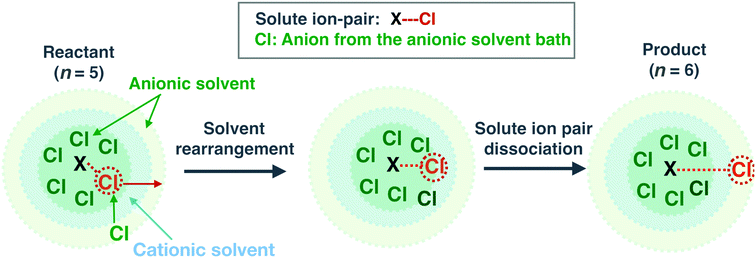Fig. 1 Different solvation shells and definition of the reactant, product, and transition states for anion exchange processes around a cation (X+) in terms of (i) the distance (r) between X+ and a select (red-circled) Cl− that together form the solute, and (ii) the number (n) of solvent Cl− (green) that are coordinating with the cation X+. Along the pathway of anion exchange driven by solvent rearrangement, the transition and product states have higher values of n than the reactant state. The values of n in this scheme are hypothetical.

For our 2-D reaction coordinate, we compute the joint probability distribution (P(r,n)) of r and n from which a 2D-free energy landscape can be obtained through the expression W(r,n) = −kBTlnP(r,n). We also define the quantity dN = 4πr2ρAP(r,n)drdn as the number of cases for which the solute X+–Cl distance is between r and r + dr and the X+–solvent Cl coordination number is between n and n + dn (ρA is the anionic number density). As required in our rate theory formalism, we define n in terms of a smooth function (continuously differentiable), f(ri);34,35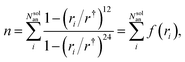(9)
where ri is the distance between X+ and the ith Cl out of the Nsolan solvent anions; r is the location of the boundary of the first solvation shell defined from the cation–anion gαβ(r) shown in Fig. S3 in the ESI.

According to Marcus theory, an anion exchange process can be described by considering the reactant (R) and product (P) states as 1D parabolic functions of n, which are extracted from W(r,n) as follows: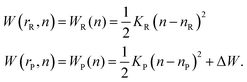(10)
In eqn (10), KR and KP are the reactant and product parabola curvatures with minima at n = nR and n = nP, respectively. ΔW is the free energy difference between the product and reactant equilibrium states (WP(nP) − WR(nR)). To obtain WR(n) and WP(n), we extract parabolically fitted slices through W(r,n) at r = rR and r = rP that represent the equilibrium cation–anion separations in reactant and product states, respectively. In our simulations, we find that rR and rP derived from the minima in W(r,n) are identical to those derived from the 1D free energy, W(r) = −kBTln(gαβ(r)). The extracted parabolas are diabatic states and cross at a particular coordination number that defines the transition state (n), representing the aforementioned anionically overcrowded state. The Marcus parabolas can couple in their overlapping region, resulting in a lower and a higher adiabatic free energy surface. As we will see in the results section, in all our cases, reactant and product minima are well-separated and the parabolas cross in the “normal region” (opposite sides). Therefore, reactant-to-product transitions can be described by the transition dynamics on the lower adiabatic free energy surface.34,35

The free energy barrier (ΔW) for the reactant-to-product transition takes a simple expression (eqn (11)) when KR = KP. In eqn (11), ΔW depends on ΔW and the solvent reorganization energy (λ = WR(nP) − WR(nR)) which is the energy cost required for changing the equilibrium reactant coordination state to the equilibrium product coordination state.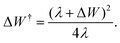(11)
Instead, when KRKP, the barrier is sensitive to the difference between these curvatures35 and there are two crossing points. The reactant-to-product transition should most-likely occur through the crossing point with the lowest barrier given by:35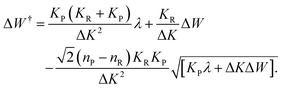(12)

Using the free energy barrier derived from Marcus theory, eqn (13)34,94 provides a simple approximation to the transition rate between reactant and product states based on Wigner's transition state theory (TST).38,39,94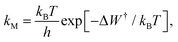(13)
where h is the Planck constant. In eqn (13) the product of a prefactor, kBT/h, and a Boltzmann factor associated with the free energy barrier account for the equilibrium flux of trajectories through the dividing surface (transition state) between the reactant and product states. However, Schenter et al.39 showed that an accurate determination of the equilibrium flux for a particular reaction coordinate generally requires the determination of the prefactor specific to that reaction coordinate. Because of this, in our calculations, we will use the recently-extended TST formulation by Roy et al.,54 where the transition rate between different states depends on the prefactor, the free energy barrier, and the transmission coefficient (representing barrier-recrossing) all of which are sensitive to the choice of the reaction coordinate. Specifically, our first estimation of anionic exchange rates will combine Marcus theory's lower adiabatic free energy surface, W(n), with Roy's TST formalism for n as given in eqn (14).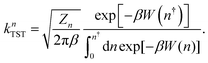(14)
Here, n is the location of the transition state (barrier) on the lower adiabatic surface, which is the same as the location of the crossing point between the two Marcus diabats, β = 1/kBT, Zn = Λ/μ, μ is the reduced mass of a select cation–anion pair, and Λ is given by:54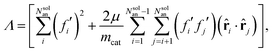(15)
where mcat is the mass of the cation. The derivative,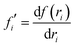, is obtained from the function, f(ri), (defined in eqn (9)) with respect to the distance between the cation and the ith solvent anion, as given in eqn (16); fj′ is identically defined for the jth solvent anion.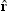i andj are the unit distance vectors pointing from the cation to the ith and jth solvent anions, respectively.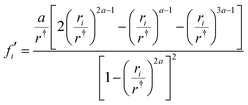(16)
In eqn (16), a = 12, and we will see in the results section that for each alkali halide salt, Zn in eqn (14) is an essentially conserved quantity that minimally fluctuates over time around its average value. Another key result will be that whereas there is no particular trend for Λ across the systems, there is a clear trend for Zn that is dominated by the value of μ. We highlight that in Roy's formulation,54 the dynamics associated with an arbitrary reaction coordinate has an associated mass-like quantity that enters the prefactor in kTST. As an example, when using n as a reaction coordinate the corresponding mass-like quantity in eqn (14) is 1/Zn with Zn = Λ/μ; instead if the reaction coordinate is defined as the interionic distance between a cation and an anion (vide infra) the mass-like quantity is μ (i.e. Zr = 1/μ). We will see in the results section that independent of the reaction coordinate (n or r), for the alkali halides in the molten state this mass-like component within the TST prefactor will become important in distinguishing their dynamical behavior.

If Marcus theory was exact, upon arrival at the crossing point of the reactant and product parabolas from the reactant equilibrium coordination state, an anion spontaneously dissociates from the paired state with the cation leading to the product coordination state. However, it is possible that there is a non-vanishing barrier along the ion pair distance at the crossing point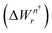, making the dissociation event nonspontaneous.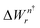can be determined by extracting a slice from W(r,n) at n = n; the first barrier on that slice provides. If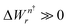, it must be accounted for as an additional barrier for the reactant-to-product transition. Furthermore, rapid fluctuation of the surrounding solvent coupled to the motion along the coordination number can cause recrossing at the crossing point, resulting in effectively slower transition rates. Such nonequilibrium solvent effect can be treated by utilizing the semiclassical approach of Landau30,95 and Zener,31 which corrects Marcus rates through the determination of the transmission coefficient (κLZ). κLZ is defined in terms of the probability (P) of reactive transitions through the crossing region and the location of the crossing region:32,34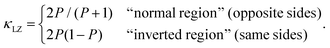(17)

Since for all the molten alkali chloride studied here the Marcus parabolas cross on opposite sides, only the “normal region” expression in eqn (17) is relevant to our study. P depends on the coupling (C) between the reactant and product parabolas and the positive traversal velocity (vn) in coordination number space at the crossing point: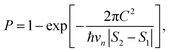(18)
where ħ = h/2π,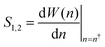are the slopes of the parabolas at the crossing point, and vn is the mean value of the traversal velocity distribution, D(v), at the crossing point obtained from the phase space trajectories of the coordination number. Our simulations showed that the velocity distribution at the crossing point has a Gaussian form: D(v) = D0exp(−v2/σn2), and therefore vn can be obtained as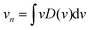. C is evaluated at n = n using the formula,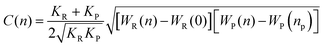, where the prefactor disappears if the reactant and product parabolas have equal curvatures.34 Note that, P is mostly dominated by the reactant–product coupling strength, C, affecting the transition rates. A stronger reactant–product coupling leads to a larger probability of reactive transition resulting in a larger transmission coefficient and a faster transition rate for two distinct Marcus parabolas that cross at the “normal region”. Now, after incorporating the additional, non-vanishing barrier at the transition state, the transmission coefficient (κLZ), and kM = knTST, the corrected Marcus rate expression (kCorrectM) takes the form of: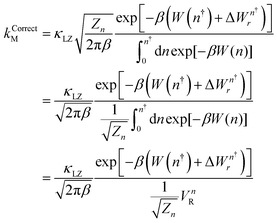(19)
At this point, we recognize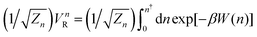as a type of mass-weighted configuration space region in coordination number which we dub “mass-weighted reactant volume” since the integral sums over the Boltzmann-weighted volume elements of coordination number (dn) in the reactant region (as gleaned from the upper limit of the integral, which is the location of the transition state, n). It will become apparent in the results section that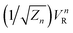plays an important role in distinguishing cationic size effects on the anion exchange rates.

A complementary analysis to the one discussed above in the case of n as a reaction coordinate can be carried out instead utilizing TST with the distance r as the reaction coordinate. This approach has been extensively used to study ion-pairing and solvent exchange kinetics,46,48–50,96–108 where nonequilibrium solvent effects are treated by computing the transmission coefficient with a variety of methods such as that of Krammer,46 the Grote–Hynes's stable state picture,48 or the reactive flux (RF) method49,50 by Chandler and Bennett. We see from eqn (20), corresponding to Roy's approach,54 that the expression is also influenced by a mass-weighted configuration space “reactant volume”,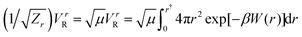, in addition to the barrier (W(r)) and the transmission coefficient (κRF).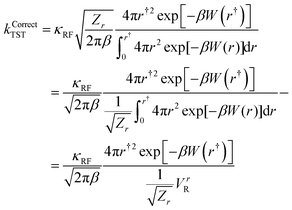(20)
Simple manipulations of the above expression result in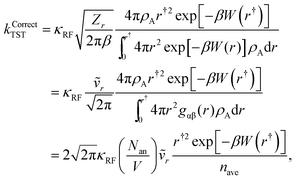(21)
where nave is the average coordination number of the cation and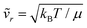is a quantity proportional to the average relative velocity between a cation and an anion given that the velocities of ions are distributed according to Maxwell–Boltzmann statistics. For this article, we have chosen to use the reactive flux method49,50 to determine the transmission coefficient κRF(t), which is a time-dependent quantity defined as: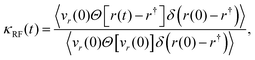(22)
where r(0) and vr(0) are respectively the initial X+–Cl distance and associated velocity at the top of the barrier (r) on the potential of mean force W(r) and Θ is a Heaviside step function. Because of the need for extensive averaging, all calculations associated with this section are for our PIM and RIM at 1100 K.

## 4 Results and discussion

This section discusses our findings on structure, diffusion and conductivity as well as possible connections between these and different rate theory models focused on anionic exchange in and out of the first cationic solvation shell.

### 4.1 Structure of molten alkali chloride salts from X-ray scattering measurements

High-energy X-ray data were collected at Brookhaven National Laboratory (NSLS-II) using the methodology described in Section 2.2. These measurements provide S(q) as defined in eqn (1), and via the transformation defined in eqn (2), the reduced pair distribution function D(r). We use both of these functions to interpret liquid structure and provide a benchmark comparison with simulation results. A key aspect in the generation of D(r) is the finite maximum q value (qmax) of the measured S(q). Early experiments had a short cutoff value that likely introduced significant truncation errors limiting the accuracy of D(r) particularly in key short-range regions. These issues are minimized by measuring S(q) up to a sufficiently high momentum transfer at which S(q) → 0. Unlike standard laboratory X-ray instruments, the high energy photons from modern synchrotron sources achieve a wide q-range at a relatively small scattering angle, significantly minimizing truncation issues and also reducing sample attenuation, multiple scattering, and other angle-dependent correction factors.11,72Fig. 2 nicely illustrates the effect that a small qmax in S(q) (and hence in the upper limit of the integral in eqn (2)) has on D(r) by comparing the old X-ray diffraction results by Takagi et al.24 with our experiments for molten KCl at 1173 K. As can be seen from the top panel, there are still noticeable oscillations in S(q) beyond ∼7 Å−1 (qmax achieved by Takagi) and a more reasonable truncation value should be q ∼ 12 Å−1. As expected, D(r) in the bottom panel of Fig. 2 shows significant differences at all ranges of r across experiments. These are mostly due to truncation differences since on the range measured by Takagi and coworkers the actual S(q) is very similar to that measured at NSLS-II. This example provides an important cautionary tale as many of the pioneering X-ray and neutron scattering experiments on molten salts (from which quantities such as coordination numbers and first neighbor solvation geometries are based) were limited due to technical constraints to small qmax values.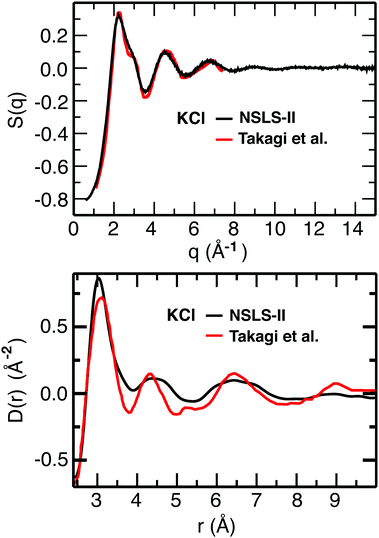Fig. 2 For KCl at 1173 K, experimental S(q) (top) and reduced pair distribution function D(r) (bottom) from this work compared to that of Takagi and coworkers from ref. 24.‡ Takagi's real-space data were generated by digitizing the pair distribution function, G(r), in Fig. 1 from ref. 24 and converting it to D(r) using the expression: D(r) = 4π*ρ0*r*G(r),72,74 where ρ0 = 0.02345 atoms per Å3.87 (G(r) = g*(r) − 1 in Takagi's notation). Takag's reciprocal-space reduced intensity data were digitized from Fig. 2 in ref. 24 from which Icoh was derived based on eqn (8) in the same article. Icoh was then used to compute S(q) based on eqn (1) in our article.

To examine whether our MD simulations can accurately describe the structure of the molten chloride salts, we computed X-ray structure functions, S(q), at different levels of theory to compare with those from the synchrotron X-ray scattering experiments. Fig. 3a–c show that across systems, simulations using each of three models (PIM, RIM, and ab initio) capture features of the experimental S(q) fairly well, but the PIM model appears to be overall slightly more accurate when comparing peak positions and intensities. This is in part because the chloride ion is significantly polarizable, because AIMD results may be sensitive to the chosen DFT flavor, and also because DFT simulations boxes are necessarily smaller than those used for the PIM and RIM simulations.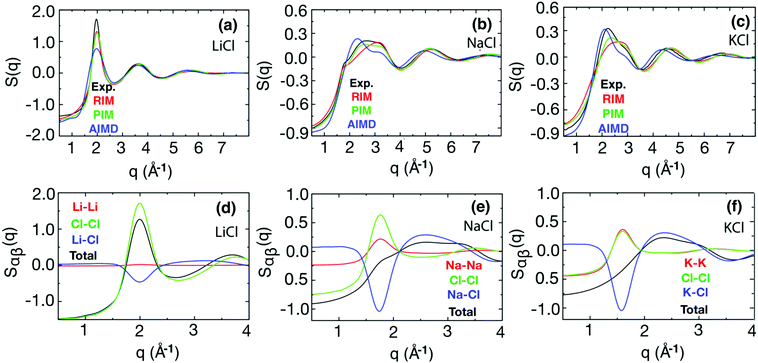Fig. 3 Comparison between our NSLS-measured and our simulated X-ray structure functions for LiCl at 958 K (a), NaCl at 1148 K (b), and KCl at 1173 K (c) and corresponding decompositions of the total structure function into cation–cation, anion–anion, and cation–anion subcomponents (partial structure functions) as defined in the ESI of ref. 69 and 70 obtained from PIM simulations for LiCl (d), NaCl (e), and KCl (f).

The interpretation of peaks in S(q) can be achieved by decomposing the function into contributions from the different ion-pair subcomponents (the partial structure functions, Sαβ(q)). Fig. 3d–f show how cation–cation, anion–anion, and cation–anion correlations contribute to the total S(q) determined from the PIM simulations. The inherent structural characteristic of molten salts is charge alternation, and its signature is a positive-going peak arising from the contributions of same-charge ions at about the same q value as a negative-going peak (also known as an antipeak109–116) resulting from spatial correlations between opposite-charge ions. For LiCl, these charge-alternation peaks and the concomitant antipeak are present around ∼2 Å−1; peaks and antipeaks corresponding to this feature move to lower q values (∼1.75 Å−1 for NaCl and ∼1.6 Å−1 for KCl) with larger cation size. This is because these peaks and the corresponding antipeak are linked to the distance between ions of the same charge alternated by ions of opposite charge which becomes larger as the cation size increases. Summing the three contributions (cation–cation, anion–anion and cation–anion) results in a prominent peak in the total S(q) for LiCl but the peak diminishes in intensity for NaCl and is completely absent for KCl. This is purely related to lack of contrast in X-ray experiments; the X-ray form factor for Li is small but that for Cl is large and the sum of intensities of the two peaks (Li–Li and Cl–Cl) are not cancelled by that of the Li–Cl antipeak at the same q value. Instead, there is essentially complete cancellation of peaks and antipeaks for KCl resulting in a missing charge alternation feature in the overall S(q).69 As is obvious from the two large (and nearly identical) peaks and the antipeak in Fig. 3f, this does not mean that there is no charge alternation in KCl but instead, that there is a poor contrast in the technique. For example, the result would be different if one was to use neutron weighting factors instead of those for X-ray. The peak at a larger q value than charge alternation is what we commonly call the “adjacency peak”.109–116 In general, this peak is associated with short-range structural interactions between nearest neighbors. In the case of salts, these are commonly opposite-charge ion interactions. This can be clearly gleaned from the similarity between the total S(q) and the cation–anion partial S(q) above ∼2 Å−1 in Fig. 3e and f. The case of LiCl is particularly interesting since there is a major weight difference in the X-ray form factors between the cation and the anion. This results in no apparent Li–Cl adjacency peak; in Fig. 3d, the black line does not look like the blue line between 2.5 and 3 Å−1.

Real space D(r) functions obtained by the Fourier transform of the total structure functions (eqn (2)) are depicted in Fig. 4a–c, and compositionally resolved partial Dαβ(r) from the PIM simulations (as defined in eqn (3)) in Fig. 4d–f. Corresponding pair distribution functions gαβ(r) are provided in Fig. S3 of the ESI. These figures show that the shortest contact ion pair distance (dominating the first peak in D(r)) is achieved for LiCl, followed by NaCl and KCl as is obviously expected from the trend in cationic sizes. It is worth noting that the reduced pair distribution function, D(r), emphasizes the large-r portion of the data and this helps highlight ionic correlations beyond short-range interactions. Partial Dαβ(r) from our MD simulations facilitate the interpretation of real-space data by showing how particular ion–ion correlations are manifested in the total D(r). As the number of electrons in the cation increases, so does the cation–cation contribution to D(r). Analogous to the case of the partial subcomponents of S(q) discussed in the previous paragraph, this is simply an issue of contrast in the X-ray technique. Overall, our results appear to indicate that simulations, particularly the less expensive classical ones, can be used for the interpretation of experimental results.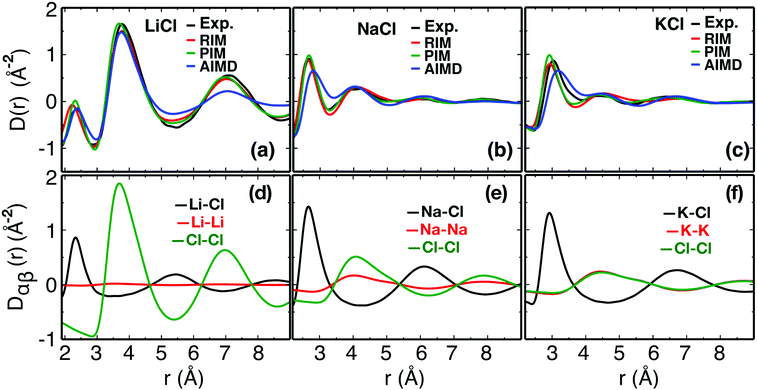Fig. 4 Comparison between our simulated and experimental D(r) functions for LiCl at 958 K, NaCl at 1148 K, and KCl at 1173 K (top row) and interpretation of different peaks in terms of the sub-components of the total D(r) obtained from the PIM simulations (bottom row) (see also pair distribution functions gαβ(r) in Fig. S3 of the ESI†).

### 4.2 Dynamical macroscopic properties

In this section, we present results on diffusivity and conductivity and discuss these in the context of several prior studies in the molten salts and ionic liquids literature.9,55,60,65,117,118Fig. 5a depicts the self-diffusion coefficients and ionic conductivities computed from the PIM and RIM simulations at 1100 K (see eqn (4)–(7)) in comparison to experimental data119,120 as a function of cationic size. Fig. 5a shows that whereas both models correctly capture the order of magnitude and trends in diffusion coefficients, there are clear differences between these. Experimental and simulation results indicate that there is a marked distinction between the diffusivity of Li+ which is high and that of Na+ and K+ which are lower and quite similar. Fig. 5c shows experimental and computational conductivities (see Table S1 in the ESI for values and error estimations). Here too there are differences across models and experiments but the trend is clear; there is a decrease in conductivity with an increase in cationic size. The reader is reminded that there is an explicit dependence on the volume in the conductivity (eqn (6)) and since the molar volume for each of these salts is different, trends in Fig. 5c must concomitantly capture the differences in molar conductivity and molar volume. We notice that trends in the molar conductivity (Fig. 5b) are much more similar to those in the diffusion coefficients, where going from NaCl to KCl changes are much more modest than going from LiCl to NaCl. We find that neither model perfectly captures the experimental trend in molar conductivity (both models show essentially flat behavior for NaCl and KCl) but models and experiments coincide in that the change in going from LiCl to NaCl is much more pronounced (about 2.65 times larger for the experiment) than in going from NaCl to KCl.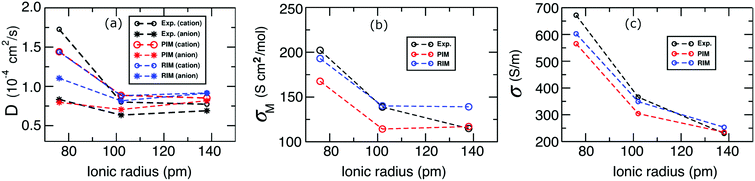Fig. 5 Variation in the diffusion coefficient, molar conductivity, and conductivity with increasing ionic radius of the cation obtained from experiments119,120 as well as the PIM and RIM for LiCl, NaCl, and KCl at 1100 K.

The ionic conductivity, is made of contributions from the collective dynamics of same- and different-charge species that goes beyond the self-diffusion dynamics of cations or anions. This can be gleaned from eqn (6) which can be expanded110 as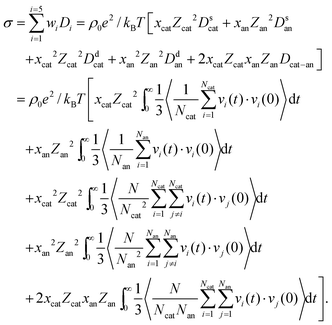(23)
In eqn (23), e is the protonic charge, Ncat and Nan are the number of cations and anions, respectively, providing the total number of ions N = Ncat + Nan. Zcat = 1 and Zan = −1 are their charge numbers and xcat = 0.5 and xan = 0.5 are their mole fractions. In eqn (23), the integrals (including the factor 1/3) represent different types of diffusion coefficients; cation–cation and anion–anion self-diffusion coefficients (Dscat and Dsan) and distinct diffusion coefficients (Ddcat and Dsan) and the distinct cation–anion diffusion coefficient (Dcat–an). Eqn (23) highlights that ionic conductivity is proportional to the weighted sum of these five different diffusion coefficients (Di), where their prefactors represent the weights (wi). Kashyap et al.118 examined the behavior of these different velocity auto- and cross-correlation functions and found that short-time ionic motion is very important to the overall ionic conductivity; they also explained that the relative motion of opposite-charge ions enhances conductivity.

Fig. 6 shows all correlation functions (Cv) as well as the weighted contributions of the different diffusion coefficients to the conductivity. As can be seen from Fig. 6, for the most part, all Cv functions decay to zero within a fraction of a picosecond (although small oscillations still persist beyond this time that contribute to their time integrals) confirming the idea that what happens at a very short time is extremely important to the overall value of the conductivity in these systems. A few things can be learned from the bottom panel in Fig. 6. First, as expected from AB-type ionic systems where there is no third neutral solvent component to act as a “momentum buffer”,118 the cation–anion contribution to the conductivity is positive. This is in contrast to what happens to ions in solution where ion-pairing reduces conductivity. Second, the behavior of LiCl across the different weighted Di functions is very different from that of NaCl and KCl which are much more similar. This is not to say that the Cv functions are similar for NaCl and KCl, but in most cases, the long-time weighted integrals are when compared to those for LiCl. This is particularly clear in the case of the cationic self contribution, but is also apparent for the anionic self and distinct contributions. In the case of LiCl the positive contribution of the anionic self term is roughly cancelled by that of its distinct contribution; since cation–anion and cation distinct contributions across the three salts are not that different, one can fairly state that it is the self diffusion component for Li+ that makes the conductivity for LiCl distinctly different from that of NaCl and KCl. Notice that distinct cation and anion contributions in Fig. 6i and j across the three salts appear to be in reverse order and in the case of NaCl and KCl these differences roughly cancel each other resulting in overall conductivities for NaCl larger than for KCl that follow the trends in Fig. 6f–h. It is now easier to see why the drop in conductivity is much larger when going from LiCl to NaCl than from NaCl to KCl. The dominating effect is simply the high self diffusivity of Li+ and the similarity in trends between Fig. 5a and b confirms this.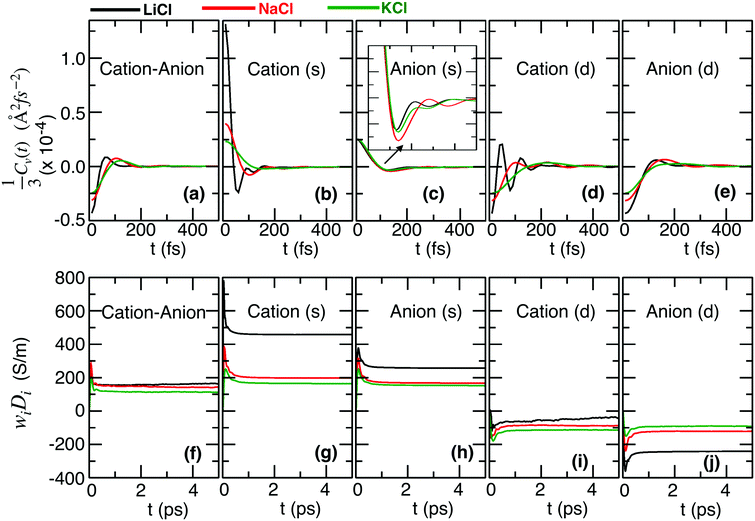Fig. 6 Various types of self (s) and distinct (d) velocity correlation functions for LiCl, NaCl, and KCl at 1100 K (a–e). The time-dependent weighted contribution of the different diffusion coefficients to the conductivity (f–j); positive contributions from the cation–anion, self cation–cation, and self anion–anion increase the conductivity, whereas cation–cation and anion–anion distinct contributions decrease conductivity. See Fig. S4 (ESI†) highlighting the short-time behavior of the weighted diffusion coefficients.

### 4.3 Rate theories of anion exchange for the alkali halides

To motivate this section and our interest in understanding solvation-shell dynamics and ion exchange processes for the alkali halide molten salts, we remind the reader that computational work by Zhang et al.117 investigated around 30 ionic liquids at various temperatures, and found that, independent of the choice of system and temperature, there was a nearly linear relation between the lifetime of the closest cation–anion neighbors and both their self-diffusion coefficient and ideal or Nernst–Einstein ionic conductivity. It is therefore reasonable to conjecture that for these molten salts, counter-ion exchange could perhaps also be taken as a proxy to understand transport properties. For this purpose, we use three different techniques that provide alternative but complementary views of the anion exchange process. The first technique is based on a combination of Marcus theory and transition state theory where the reaction coordinate is the coordination number n of an alkali chloride ion pair as discussed in the methods section, the second is the more traditional transition state theory where the distance between a cation and an anion is taken as the reaction coordinate, and the third one is based on the survival probability correlation function.

The first two methods require knowledge of the free energies, W(r), W(r,n), and W(n), which are presented in Fig. 7a–g for the PIM simulations at 1100 K. Fig. S5 in the ESI provides a comparison between the PIM and RIM results, indicating a good agreement between them. The reader is reminded that n in the y-axis of W(r,n) is defined as the number of counter-anions to a central alkali metal ion not counting the special anion defined to be its pair (the progression from reactant to transition and finally product states as well as the definition of n are depicted in Fig. 1). This theoretical construct is used so that n is different (smaller) in the reactant state than in the product state even though the actual number of Cl ions surrounding the cation is the same.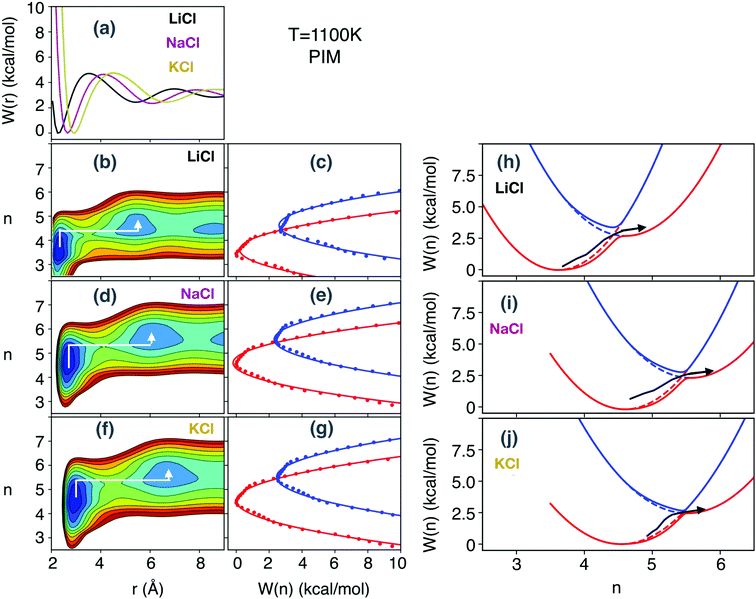Fig. 7 One-dimensional free energy along the solute cation–anion distances (W(r)) (a) and their extensions to two-dimensions (W(r,n)) by including the coordination number of the solute cation (n) with the solvent anions (evenly spaced (1 kcal mol−1) contours between 0 and 10 kcal mol−1) (b, d and f). White arrows on W(r,n) display the Marcus pathways of ion-pair dissociation. Slices through W(r,n) at r = rR and at r = rP (dotted lines) and their parabolic fits (solid lines) represent reactant and product states in coordination number space used in Marcus theory (c, e and g). Using Marcus theory, the ion pair dissociation and anion exchange mechanism around a cation in molten salts can be described as the adiabatic traversal of the crossing point of the Marcus parabolas (dashed red and blue, respectively) that couple to generate lower and higher free energy surfaces (solid red and blue, respectively) (h, i and j). These results are from PIM simulations at 1100 K for LiCl, NaCl, and KCl, whereas other cases are discussed in the ESI.†

For the different salts, W(r) clearly distinguishes the equilibrium distances corresponding to the contact ion pair (rR) in the reactant state (R) and the solvent-separated ion pair (rP) in the product state (P). These equilibrium distances systematically increase going from LiCl to NaCl to KCl. However, we notice that barriers along the solute cation–anion distance are very similar (∼4.6–4.8 kcal mol−1) for all the salts and are significantly higher than the thermal energy at 1100 K (2.18 kcal mol−1). Overcoming this barrier makes ion-pair dissociation an activated process. Of course, a 1D-free energy hides the fact that barriers can be circumvented if pathways are considered in multi-dimensions such as those shown by the white arrows on the 2D-free energy landscapes in Fig. 7b–f. Physically, the white arrows highlight three mechanistic steps: first, solvent-rearrangement induces activation in coordination number (n) leading to anionic overcrowding (notice that while at the transition state n < nP, the actual number of anions surrounding the cation including the one considered as the ion pair is larger than in either of the wells); second, the overcrowding reduces the barrier along the solute cation–anion distance (r), and third the dissociation of the solute ion-pair along r takes place where the solvent anion takes the place of the solute anion as the product state. Cuts on the 2D-free energy surface at r = rR and r = rP (the diabats) in Fig. 7c–g have minima that are well separated and cross in the “normal region” (opposite sides) in the Marcus theory sense. Lower and higher adiabatic free energy curves can be derived from these as discussed in the methods section and are shown in Fig. 7h–j.

Our first method to study rates of chloride exchange across the family of alkali chlorides, is the hybrid Marcus-TST approach associated with eqn (19). All essential parameters entering this equation in the case of the PIM and RIM are provided in Table 1 (see Table S2 in the ESI for the remaining parameters including those defining Marcus parabolas and their couplings and traversal velocities at the crossing point). τ values in Fig. 8 (labeled Marcus), which are the reciprocal of the exchange rate, show a clear trend of increasing exchange times with an increase in cation size. There are several factors contributing to this; a large contributor is what we defined in the methods section as the mass-weighted “reactant volume” in coordination number space,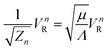. The reader should notice that for a given system, Zn = Λ/μ is essentially a conserved quantity (its value as a function of time is shown in Fig. S6 of the ESI), which has complex contributions of the solvent anions incorporated in Λ (see eqn (15)). However, Λ is almost the same for all three salts (considering their error bars) as shown in Table 1. Thus, the trend in Zn is mostly dominated by μ, therefore by the mass of the cation (as all three salts have the same anion). On the other hand, VnR does not show any specific trend (see Table 1), which leads to the conclusion that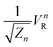is predominantly governed by the mass of the cation. For the PIM,a little more than doubles (the ratio is ∼2.19) in going from LiCl (∼0.36) to KCl (∼0.79), and since its role is multiplicative (see eqn (19)) so does the value of τ. The second most important factor in the trend for τ is the Landau–Zener transmission coefficient which is larger for LiCl (κLZ ∼ 0.97–0.98) than for NaCl (κLZ ∼ 0.91–0.94) and KCl (κLZ ∼ 0.64–0.80). For the PIM, in going from LiCl to KCl, its effect is to increase τ by about 52%. The large values of κLZ, particularly for LiCl and NaCl, are indicative of a weak coupling to the solvent bath and small nonequilibrium solvent effects for n. Yet, differences in κLZ are significant in distinguishing LiCl from KCl when it comes to the anion exchange rate. The last factor that contributes to differences in the anion exchange rate is the barrier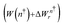, which is slightly larger for KCl (4.8–4.9 kcal mol−1) than for NaCl and LiCl (4.4–4.6 kcal mol−1). The effect from the barrier (see the term,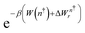in Table 1) in differenciating the cations is small, on the order of 9% in going from LiCl to KCl for the PIM.

Table 1 Important factors in the Marcus-TST hybrib method that uses n as the reaction coordinate
LiCl NaCl KCl
μ (g mol−1) 5.81 13.95 18.59
PIM(kcal mol−1) 2.45 2.66 2.68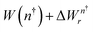(kcal mol−1) 4.60 4.63 4.880.12 0.12 0.11
κ LZ 0.97 0.91 0.64
Λ (nm−2) 61.93 ± 5.05 63.61 ± 3.49 57.63 ± 2.97
Z n (nm−2 g−1 mol) 10.66 ± 0.78 4.56 ± 0.25 3.10 ± 0.16
V n R 1.18 1.42 1.38(nm g1/2 mol−1/2) 0.36 ± 0.01 0.66 ± 0.02 0.79 ± 0.02
RIM(kcal mol−1) 2.09 2.35 2.51(kcal mol−1) 4.39 4.38 4.820.13 0.13 0.11
κ LZ 0.98 0.94 0.80
Λ (nm−2) 60.31 ± 4.60 61.10 ± 3.63 54.50 ± 3.16
Z n (nm−2 g−1 mol) 10.38 ± 0.79 4.38 ± 0.26 2.93 ± 0.17
V n R 0.98 1.27 1.16(nm g1/2 mol−1/2) 0.30 ± 0.01 0.61 ± 0.02 0.68 ± 0.02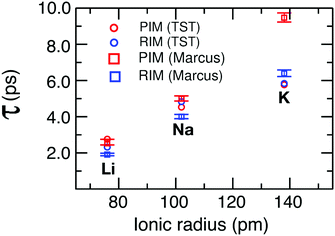Fig. 8 τ (the reciprocal of the rate of anion exchange) as a function of ionic radius from MD simulations for LiCl, NaCl, and KCl at 1100 K derived from our Marcus-TST approach based on n and the TST based on r as reaction coordinates.

The reader is reminded that the conductivity across the family of alkali chlorides in Fig. 5c shares the decreasing trend shown by the exchange rate, which is inverse to the increasing trend for τ shown in Fig. 8. Furthermore, we note that as the cationic mass (size) and concomitantly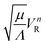increase, so does the macroscopic volume across the family of molten salts at the same temperature and pressure. In going from LiCl to KCl, this increase in the overall macroscopic volume has an explicit effect on their conductivity. This can be clearly gleaned from the prefactor in eqn (6) and the fact that conductivity and molar conductivity have different trends. The latter, which loses this prefactor, flattens out significantly as a function of cationic size, particularly when going for NaCl to KCl. It is therefore notable that an increase in cationic mass/size consistent with an increase in the macroscopic sample volume goes along with a decrease in conductivity as well as in the anion exchange rate.

The second TST approach that we used to study anion exchange rates and derive τ (see Fig. 8 labeled TST), also follows the formulation by Roy et al.54 but in this case for r as the reaction coordinate. The expression for the anion exchange rate is given in eqn (21) for which the relevant quantities are W(r) (shown in Fig. 7a), the reactive flux transmission coefficients (shown in Fig. 9 for the PIM simulation and in Fig. S7 in the ESI for the RIM simulation), the quantity r linked to the thermal average relative velocity between the cation and the anion, the volume of the sample, and the average coordination number, nave, for the different cations (all provided in Table 2).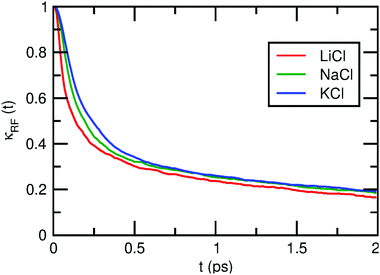Fig. 9 For the three molten salts at 1100 K, time-dependent transmission coefficients (κRF(t)) determined from the PIM simulations at 1100 K using the reactive flux method showing strong interionic distance-solvent coupling. The values of κRF used in the expression for τ are derived from the average of κRF(t) in the region between 1 and 2 ps (same protocol for the RIM in Fig. S7, ESI†).
Table 2 Important factors in the TST method that uses r as the reaction coordinate
LiCl NaCl KCl
μ (g mol−1) 5.81 13.95 18.59
v r (nm ps−1) 1.26 0.81 0.70
PIM
κ RF 0.20 0.22 0.22
V (nm3) 42.54 53.89 71.46
n ave 4.34 5.16 5.18
r (nm) 0.36 0.41 0.45
W(r) (kcal mol−1) 4.83 4.65 4.75
eβW(r) 0.11 0.12 0.11
RIM
κ RF 0.21 0.21 0.22
V (nm3) 45.87 57.63 78.82
n ave 3.90 4.82 4.89
r (nm) 0.36 0.41 0.45
W(r) (kcal mol−1) 4.60 4.61 4.58
eβW(r) 0.12 0.12 0.12

In this case, the analysis appears to be much simpler. For all three liquids, κRF is essentially the same; the structural component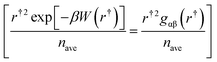would make the rate of anion exchange for KCl > NaCl > LiCl, but both the anionic number density, Nan/V, and r contribute to the opposite trend making the anion exchange significantly faster for systems with a smaller and lighter cation. Since Nan is the same for all systems, the trend observed in anion exchange rates appear to be simply determined by μ (through r), and the volumetric differences across samples. This correlates well with findings in Fig. 5 where Li has the faster diffusivity, conductivity, and molar conductivity, whereas for NaCl and KCl the larger conductivity for the former is majorly influenced by the overall sample volume.

Notice that interpretation on how the similar values across theories for τ in Fig. 8 are arrived at can be quite different when the analysis is based on r vs. n as reaction coordinates. For example, nonequilibrium solvent effects depends on how a reaction coordinate couples with the solvent bath, and when n is the reaction coordinate, those are reflected in κLZ. Values for κLZ hinted at a relatively weak coupling to the solvent, but the numerical value differences across salts made a very significant difference to the observed τ values as we went from LiCl to KCl. Previous studies on aqueous electrolytes98–108 indicate that the distance coordinate strongly couples with the solvent resulting in very small transmission coefficients (≪1). Herein, we also find the interionic distance-solvent bath coupling to be strong and affecting equally all three molten salts, since the reactive flux transmission coefficients are small and similar for all of them (∼0.20–0.22). Thus, while nonequilibrium solvent effects on the distance coordinate equally influence the anion exchange rates for all salts, the same effects on n as a reaction coordinate can slow down the rate a small amount for NaCl but a significant amount for KCl when compared to LiCl. It is therefore clear that the very nature of the nonequilibrium solvent effects strongly depends on our choice of reaction coordinate and the specific rate theory method used, and therefore, it is not an unambiguous factor. The reader is reminded that, for the TST approach where r is the reaction coordinate, the barrier for anion exchange is almost identical (W(r) ∼ 4.6–4.8 kcal mol−1) across the family of alkali halide salts. The barrier enters the structural factor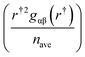through the value of the pair distribution function at r, and the overall contribution of this factor is opposite to the actual trend of anion exchange. In the Marcus-TST approach where n was the reaction coordinate, the total barrier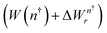was also quite similar (∼4.4–4.9 kcal mol−1) across systems. Thus, consistent across the two methods so far discussed, barrier effects do not determine the cationic size effect on the rates of anion exchange. This leads us to the overall conclusion that μ (and therefore the mass of the cation) is an important factor in both rate theories, while solvent effects are important to the Marcus-TST approach based on n, and differences in molar volume of the melt are important in the TST approach based on r.

As a final and more direct way to interrogate the process of anion exchange across our systems, we utilized the method proposed by Impey, Madden, and McDonald (IMM) to compute the residence time of a solvent molecule (in our case the chlorides) in the first solvation shell of the alkali metal cations.121 The IMM method determines the survival probability (P(t,t + δt,t*)) of a anion in the first solvation shell of a cation where P is assigned a value of 1 when an anion is found in the first solvation shell at both times t and t + δt and during this time interval the anion does not leave the shell for any continuous period of time greater than t*; otherwise, P is assigned a value of 0. Numerically, in the condensed phase, particles at an artificial boundary are constantly recrossing it, but this does not mean that they have left the first solvation shell. This is why a tolerance value t* is defined to treat unsuccessful recrossing, i.e., when anions cross the boundary of the first solvation shell but rapidly return to the shell. Obviously the choice of t* will change P(t,t + δt,t*), but sensible values should keep trends unmodified. The residence time of the ith anion is obtained from the normalized time-correlation function of the survival probability

 C(t,t*) = 〈Pi(t,t + δt,t*)〉i,t/〈P(t,t,t*)〉i,t. (24)
Here 〈…〉i,t indicates averaging over ion pairs and time. We computed C(t,t*) for a range of t* (=100 fs–1 ps) making sure that t* represented only short, transient escapes of anions. In other words, we chose t* to be significantly smaller than the actual anion exchange time derived from TST and Marcus theory. Fig. 10a and c depict C(t,t*) obtained for t* = 500 fs at 1100 K in the case of the PIM and RIM respectively. Fig. 10b and d show that whereas the choice of t* affects the value of the escape times, trends are consistently the same. We analyzed our results by applying a bi-exponential fit to C(t,t*); the fit shows a fast initial (femtoseconds) component followed by a second component in the picosecond time scale and we associate this second component with the exchange dynamics resolved by the prior two rate theory methods. The escape times presented in Fig. 10 show that anion exchange is faster for LiCl than for NaCl, which is again faster but comparable to that for KCl. While the observation is true for both simulation models, escape times are more discernible between NaCl and KCl for the RIM model. It is noticeable from Fig. 10 and 8 that except for KCl the escape times predicted by the IMM method are slightly longer than the chloride exchange timescales predicted by the Marcus and TST methods.Fig. 10 (a and c) Survival probability correlation functions for t* = 0.5 ps at 1100 K; circles are simulation results and solid lines are bi-exponential fits. (b and d) Anionic escape times derived from the fits as a function of t*. (b and d) Show that independent of the model and the choice of t*, faster chloride escape is observed for LiCl than for NaCl and KCl. This is the same trend observed in Fig. 8 for τ.

## 5 Conclusions

We have studied the liquid structure, diffusion and conductivity as well as interpreted the dynamics of anion exchange across the family of alkali chlorides in the molten state. Our X-ray scattering and simulations using different techniques emphasize that the main structural feature for these systems is charge alternation. However, when comparing S(q) across these systems one finds that the lowest q-peak has a different origin for LiCl and KCl. In the case of LiCl the peak is truly a positive–negative charge alternation feature, whereas cancellation of peaks and antipeaks completely vanishes the charge alternation feature for KCl. A peak to the right (higher q) of the charge alternation peak is normally associated with the adjacent structural correlations between cations and anions, except that this peak in the case of LiCl is missing since the X-ray form factor for Li+ is so small. Both the absence of a charge alternation peak in the case of KCl and the fact that the adjacency peak is missing in the case of LiCl are simply a consequence of poor contrast in the technique, and not due to a fundamental structural difference in the melt. This finding highlights the importance of computer simulations in explaining the origin of the different S(q) features, without which, these assignments would have been much hard to accurately make. In general, the agreement between experiment and simulations both in real space and reciprocal space is satisfactory giving credence to predictions and analysis based on simulations. However, based on S(q) and D(r), it would appear that further improvements could be made to models for KCl.

The experimental and computational conductivity of these systems decreases with increasing cationic size. Two factors contribute to this decrease, the first one is the very high self contribution of Li+ compared to other ions and the second is the change in molar volume across the salts. If one corrects for the change in volume by focusing instead on the molar conductivity, there is still a significant drop in going from LiCl to NaCl, but the experimental change in going from NaCl to KCl is small and computationally there is essentially no change in molar conductivity between these two. The same type of trend is observed when considering the diffusion coefficients instead of the molar conductivity.

We used three different approaches to understand the time scale and mechanisms for chloride exchange (or escape in the case of the IMM technique) across the family of cations. In all cases, we found that consistent with the trends in conductivity, the reciprocal rate of anion exchange, τ, is larger for the larger cations. Different reaction coordinates and methods provide unique perspectives to the process; for example, the solvent ions couple strongly to the inter-ionic distance but less so to the coordination number. Whereas anion exchange is definitively an activated process, barriers to anion exchange do not appear to determine trends of exchange. The mass of the cation and solvent effects are salient factors in the Marcus-TST approach based on n whereas the mass of the cation and the overall sample volume are important in the TST approach based on r. While none of the methods are superior to any other-they all generate reasonably consistent τ values bolstering confidence in our analysis, the TST approach based on r appears to provide the most intuitive link to simple factors (the cation size/mass and the sample volume) that directly correlate with trends for the conductivity of the different melts.

In the future, it may be interesting to study mixtures of these salts, particularly the low-melting eutectics which are very relevant to technology applications. We suspect that at these lower temperatures there could be a crossover between the high-temperature dynamics (where free energy barriers are not fundamentally important in defining anion exchange) and the lower-temperature regime in which free-energetics may be all that matters. We have mentioned earlier that whereas lithium has a very high mobility in molten LiCl, when mixed in a salt with a larger cation such as KCl this is reduced significantly. This phenomenon is also common when a small cation such as Li+ is mixed in an room temperature ionic liquid; in this case it is understood that the hard Li+ cation becomes an integral part of the ionic liquid charge network stiffening it and causing an increase in viscosity. How this happens with high temperature molten salts is something we would like to explore.

## Conflicts of interest

There are no conflicts to declare.

## Acknowledgements

This work was supported as part of the Molten Salts in Extreme Environments (MSEE) Energy Frontier Research Center, funded by the U.S. Department of Energy Office of Science. BNL and ORNL operate under DOE contracts DE-SC0012704 and DE-AC05-00OR22725, respectively. The project used resources of the Oak Ridge Leadership Computing Facility at the Oak Ridge National Laboratory, supported by the Office of Science of the U.S. Department of Energy under contract no. DE-AC05-00OR22725. The 28-ID-1 beamline of the National Synchrotron Light Source II was used, which is a U.S. Department of Energy (DOE) Office of Science User Facility operated for the DOE Office of Science by Brookhaven National Laboratory under Contract No. DE-SC0012704. HW, YZ and EJM acknowledge the computational resources of Notre Dame's Center for Research Computing. FW, SS and CJM acknowledge the computational resources at the University of Iowa high performance computing facility. MSEE work at Iowa and Notre Dame was supported via subcontract from Brookhaven National Laboratory. This manuscript has been authored in part by UT-Battelle, LLC, under contract DE-AC05-00OR22725 with the US Department of Energy (DOE). The US government retains and the publisher, by accepting the article for publication, acknowledges that the US government retains a nonexclusive, paid-up, irrevocable, worldwide license to publish or reproduce the published form of this manuscript, or allow others to do so, for US government purposes. DOE will provide public access to these results of federally sponsored research in accordance with the DOE Public Access Plan (http://energy.gov/downloads/doe-public-access-plan).

## Notes and references

1. F. Lantelme and H. Groult, Molten salts chemistry, from lab to applications, Elsevier, 1st edn, 2013 Search PubMed.
2. L. Wagner, in Future Energy, ed. T. M. Letcher, Elsevier, Boston, 2nd edn, 2014, pp. 613–631 Search PubMed.
3. M. W. Rosenthal, P. R. Kasten and R. B. Briggs, Nuclear Applications and Technology, 1970, vol. 8, pp. 107–117 Search PubMed.
4. D. F. Williams and K. T. Clarno, Nucl. Technol., 2008, 163, 330–343 CrossRef CAS.
5. Y. Sakamura, T. Hijikata, K. Kinoshita, T. Inoue, T. Storvick, C. Krueger, J. Roy, D. Grimmett, S. Fusselman and R. Gay, J. Alloys Compd., 1998, 271–273, 592–596 CrossRef CAS.
6. J. Willit, W. Miller and J. Battles, J. Nucl. Mater., 1992, 195, 229–249 CrossRef CAS.
7. A. Abbasalizadeh, L. Teng, S. Seetharaman, J. Sietsma and Y. Yang, in Rare Earths Industry, ed. I. B. D. Lima and W. L. Filho, Elsevier, Boston, 2016, pp. 357–373 Search PubMed.
8. U.S. Department of Energy Strategic Plan, U.S. Department of Energy: Washington D.C., 2011, DOI: 10.2172/1021847.
9. J. Wang, J. Wu, Z. Sun, G. Lu and J. Yu, J. Mol. Liq., 2015, 209, 498–507 CrossRef CAS.
10. J. Wu, J. Wang, H. Ni, G. Lu and J. Yu, J. Mol. Liq., 2018, 253, 96–112 CrossRef CAS.
11. T. Egami and S. Billinge, Underneath the Bragg Peaks, Elsevier, 2nd edn, 2012, vol. 16 Search PubMed.
12. A.-L. Rollet and M. Salanne, Annu. Rep. Prog. Chem., Sect. C: Phys. Chem., 2011, 107, 88–123 RSC.
13. P. Ballone, G. Pastore and M. P. Tosi, J. Chem. Phys., 1984, 81, 3174–3180 CrossRef CAS.
14. R. L. McGreevy, Experimental Studies of the Structure and Dynamics of Molten Alkali and Alkaline Earth Halides, Academic Press, 1987, vol. 40, pp. 247–325 Search PubMed.
15. G. M. Abernethy, M. Dixon and M. J. Gillan, Philos. Mag. B, 1981, 43, 1113–1123 CAS.
16. M. Dixon and M. J. Gillan, Philos. Mag. B, 1981, 43, 1099–1112 CAS.
17. M. Revere and M. P. Tosi, Rep. Prog. Phys., 1986, 49, 1001–1081 CrossRef.
18. R. L. McGreevy and M. A. Howe, J. Phys.: Condens. Matter, 1989, 1, 9957–9962 CrossRef CAS.
19. M. A. Howe and R. L. Mcgreevy, Philos. Mag. B, 1988, 58, 485–495 CAS.
20. F. G. Edwards, J. E. Enderby, R. A. Howe and D. I. Page, J. Phys. C: Solid State Phys., 1975, 8, 3483–3490 CrossRef CAS.
21. S. Biggin and J. E. Enderby, J. Phys. C: Solid State Phys., 1982, 15, L305–L309 CrossRef CAS.
22. E. W. J. Mitchell, P. F. J. Poncet and R. J. Stewart, Philos. Mag., 1976, 34, 721–732 CrossRef CAS.
23. J. Locke, S. Messoloras, R. J. Stewart, R. L. McGreevy and E. W. J. Mitchell, Philos. Mag. B, 1985, 51, 301–315 CAS.
24. R. Takagi, H. Ohno and K. Furukawa, J. Chem. Soc., Faraday Trans. 1, 1979, 75, 1477–1486 RSC.
25. H. A. Levy, P. A. Agron, M. A. Bredig and M. D. Danford, Ann. N. Y. Acad. Sci., 1960, 79, 762–780 CrossRef CAS.
26. M. R. Palacn, Chem. Soc. Rev., 2009, 38, 2565–2575 RSC.
27. B. Dunn, H. Kamath and J.-M. Tarascon, Science, 2011, 334, 928–935 CrossRef CAS PubMed.
28. R. A. Marcus, Annu. Rev. Phys. Chem., 1964, 15, 155–196 CrossRef CAS.
29. R. A. Marcus and N. Sutin, Biochim. Biophys. Acta, 1985, 811, 265–322 CrossRef CAS.
30. L. D. Landau, Phys. Z. Sowjetunion, 1932, 2, 46 CAS.
31. C. Zener, Proc. R. Soc. London, 1932, 137, 696–702 Search PubMed.
32. M. D. Newton and N. Sutin, Annu. Rev. Phys. Chem., 1984, 35, 437–480 CrossRef CAS.
33. S. U. M. Khan and Z. Y. Zhou, J. Chem. Phys., 1990, 93, 8808–8815 CrossRef CAS.
34. S. Roy, M. D. Baer, C. J. Mundy and G. K. Schenter, J. Chem. Theory Comput., 2017, 13, 3470–3477 CrossRef CAS PubMed.
35. S. Roy, M. Galib, G. K. Schenter and C. J. Mundy, Chem. Phys. Lett., 2018, 692, 407–415 CrossRef CAS.
36. S. Roy and V. S. Bryantsev, J. Phys. Chem. B, 2018, 122, 12067–12076 CrossRef CAS PubMed.
37. H. Eyring, Trans. Faraday Soc., 1938, 34, 41–48 RSC.
38. E. Wigner, Trans. Faraday Soc., 1938, 34, 29–41 RSC.
39. G. Schenter, B. C. Garrett and D. G. Truhlar, J. Chem. Phys., 2003, 119, 5828–5833 CrossRef CAS.
40. E. Guàrdia, R. Rey and J. A. Padró, Chem. Phys., 1991, 155, 187–195 CrossRef.
41. R. Rey and E. Guardia, J. Phys. Chem., 1992, 96, 4712–9387 CrossRef CAS.
42. D. G. Truhlar, W. L. Hase and J. T. Hynes, J. Phys. Chem., 1983, 87, 2664 CrossRef CAS.
43. B. Peters, Reaction rate theory and rare events, Elsevier Science, Oxford, 2016 Search PubMed.
44. E. Pollak and P. Talkner, Chaos, 2005, 15, 026116 CrossRef PubMed.
45. D. G. Truhlar and B. C. Garrett, Annu. Rev. Phys. Chem., 1984, 35, 159–189 CrossRef CAS.
46. H. Kramers, Physica, 1940, 7, 284–304 CrossRef CAS.
47. E. Pollak, J. Chem. Phys., 1986, 85, 865 CrossRef CAS.
48. R. F. Grote and J. T. Hynes, J. Chem. Phys., 1980, 73, 2715 CrossRef CAS.
49. C. H. Bennett, Algorithms for chemical computations, ACS Symposium Series, American Chemical Society, Washington, D.C., USA, 1977 Search PubMed.
50. D. Chandler, J. Chem. Phys., 1978, 68, 2959–2970 CrossRef CAS.
51. D. G. Truhlar, B. C. Garrett and S. J. Klippenstein, J. Phys. Chem., 1996, 100, 12771 CrossRef CAS.
52. C. Dellago, P. G. Bolhuis and P. L. Geissler, Adv. Chem. Phys., 2002, 123, 1 CAS.
53. R. G. Mullen, J. E. Shea and B. Peters, J. Chem. Theory Comput., 2014, 10, 659 CrossRef CAS PubMed.
54. S. Roy, M. D. Baer, C. J. Mundy and G. K. Schenter, J. Phys. Chem. C, 2016, 120, 7597–7605 CrossRef CAS.
55. M.-M. Walz and D. van der Spoel, J. Phys. Chem. C, 2019, 123, 25596–25602 CrossRef CAS.
56. M.-M. Walz and D. van der Spoel, Phys. Chem. Chem. Phys., 2019, 21, 18516–18524 RSC.
57. Y. Ishii, S. Kasai, M. Salanne and N. Ohtori, Mol. Phys., 2015, 113, 2442–2450 CrossRef CAS.
58. J. Wang, Z. Sun, G. Lu and J. Yu, J. Phys. Chem. B, 2014, 118, 10196–10206 CrossRef CAS PubMed.
59. J. Wang and C.-L. Liu, J. Mol. Liq., 2019, 273, 447–454 CrossRef CAS.
60. B. Morgan and P. A. Madden, J. Chem. Phys., 2004, 120, 1402–1413 CrossRef CAS PubMed.
61. A. Bengtson, H. O. Nam, S. Saha, R. Sakidja and D. Morgan, Comput. Mater. Sci., 2014, 83, 362–370 CrossRef CAS.
62. M. Salanne, C. Simon, P. Turq and P. A. Madden, J. Phys. Chem. B, 2007, 111, 4678–4684 CrossRef CAS PubMed.
63. M. C. C. Ribeiro, J. Phys. Chem. B, 2003, 107, 4392–4402 CrossRef CAS.
64. O. Alcaraz and J. Trullàs, J. Chem. Phys., 2000, 113, 10635–10641 CrossRef CAS.
66. J. Wu, H. Ni, W. Liang, G. Lu and J. Yu, Comput. Mater. Sci., 2019, 170, 109051 CrossRef CAS.
67. I. Okada, Z. Naturforsch., A: Phys. Sci., 1987, 42, 21–28 CAS.
68. I. Okada, R. Takagi and K. Kawamura, Z. Naturforsch., A: Phys. Sci., 1980, 35, 493–499 Search PubMed.
69. F. Wu, S. Roy, A. S. Ivanov, S. K. Gill, M. Topsakal, E. Dooryhee, M. Abeykoon, G. Kwon, L. C. Gallington and P. Halstenberg, et al. , J. Phys. Chem. Lett., 2019, 10, 7603–7610 CrossRef CAS PubMed.
70. F. Wu, S. Sharma, S. Roy, P. Halstenberg, L. C. Gallington, S. M. Mahurin, S. Dai, V. S. Bryantsev, A. S. Ivanov and C. J. Margulis, J. Phys. Chem. B, 2020, 124, 2892–2899 CrossRef CAS PubMed.
71. M. Chemla and I. Okada, Electrochim. Acta, 1990, 35, 1761–1776 CrossRef CAS.
72. H. E. Fischer, A. C. Barnes and P. S. Salmon, Rep. Prog. Phys., 2006, 69, 233–299 CrossRef CAS.
73. Q. Xiangyun, J. W. Thompson and S. J. L. Billinge, J. Appl. Crystallogr., 2004, 37, 678 CrossRef.
74. D. A. Keen, J. Appl. Crystallogr., 2001, 34, 172–177 CrossRef CAS.
75. J. VandeVondele, F. Mohamed, M. Krack, J. Hutter, M. Sprik and M. Parrinello, J. Chem. Phys., 2005, 122, 014515 CrossRef PubMed.
76. M. Born and J. E. Mayer, Z. Phys., 1932, 75, 1–18 CrossRef CAS.
77. J. E. Mayer, J. Chem. Phys., 1933, 1, 270 CrossRef CAS.
78. F. G. Fumi and M. P. Tosi, J. Phys. Chem. Solids, 1964, 25, 31–43 CrossRef CAS.
79. M. P. Tosi and F. G. Fumi, J. Phys. Chem. Solids, 1964, 45–52 CrossRef CAS.
80. N. Ohtori, M. Salanne and P. A. Madden, J. Chem. Phys., 2009, 130, 104507 CrossRef PubMed.
81. M. S. Yoshiki Ishii, S. Kasai and N. Ohtori, Mol. Phys., 2015, 113, 2442–2450 CrossRef.
82. S. Plimpton, J. Comput. Phys., 1995, 117, 1–19 CrossRef CAS.
83. J. Hutter, M. Iannuzzi, F. Schiffmann and J. VandeVondele, Wiley Interdiscip. Rev.: Comput. Mol. Sci., 2013, 4, 15–25 Search PubMed.
84. S. Nosé, J. Chem. Phys., 1984, 81, 511–519 CrossRef.
85. S. Nosé, Mol. Phys., 1984, 52, 255 CrossRef.
86. W. Shinoda, M. Shiga and M. Mikami, Phys. Rev. B: Condens. Matter Mater. Phys., 2004, 69, 134103 CrossRef.
87. G. J. Janz, J. Phys. Chem. Ref. Data, 1988, 17, 2 Search PubMed.
88. G. J. Martyna, M. L. Klein and M. Tuckerman, J. Chem. Phys., 1992, 97, 2635 CrossRef.
89. Y. K. Zhang and W. T. Yang, Phys. Rev. Lett., 1998, 80, 890 CrossRef CAS.
90. J. VandeVondele and J. Hutter, J. Chem. Phys., 2007, 127, 114105 CrossRef PubMed.
91. S. Grimme, J. Antony, S. Ehrlich and H. Krieg, J. Chem. Phys., 2010, 132, 154104 CrossRef PubMed.
92. I.-C. Yeh and G. Hummer, J. Phys. Chem. B, 2004, 108, 15873–15879 CrossRef CAS.
93. Y. Zhang, A. Otani and E. J. Maginn, J. Chem. Theory Comput., 2015, 11, 3537–3546 CrossRef CAS PubMed.
94. G. K. Schenter, B. C. Garrett and D. G. Truhlar, J. Phys. Chem. B, 2001, 105, 9672 CrossRef CAS.
95. L. D. Landau, Phys. Z. Sowjetunion, 1932, 1, 88 CAS.
96. D. Truhlar, Variational transition state theory and multidimensional tunneling for simple and complex reactions in the gas phase, solids, liquids, and enzymes, Isotope effects in chemistry and biology, Marcel Dekker Inc., New York, 2006, pp. 579–619 Search PubMed.
97. B. Peters, Chem. Phys. Lett., 2012, 554, 248–253 CrossRef CAS.
98. H. V. R. Annapureddy and L. X. Dang, J. Phys. Chem. B, 2014, 118, 8917 CrossRef CAS PubMed.
99. H. V. R. Annapureddy and L. X. Dang, J. Phys. Chem. B, 2014, 118, 7886 CrossRef CAS PubMed.
100. L. X. Dang and H. V. R. Annapureddy, J. Chem. Phys., 2013, 139, 084506 CrossRef PubMed.
101. K. Hermansson and M. Wojcik, J. Phys. Chem. B, 1998, 102, 6089 CrossRef CAS.
102. D. M. Wilkins, D. E. Manolopoulos and L. X. Dang, J. Chem. Phys., 2015, 142, 064509 CrossRef PubMed.
103. S. Roy and L. X. Dang, Chem. Phys. Lett., 2015, 628, 30–34 CrossRef CAS.
104. R. Rey and J. T. Hynes, J. Phys.: Condens. Matter, 1996, 8, 9411 CrossRef CAS.
105. D. Spangberg, M. Wojcik and K. Hermansson, Chem. Phys. Lett., 1997, 276, 114 CrossRef CAS.
106. L. X. Dang, J. Phys. Chem. C, 2014, 118, 29028 CrossRef CAS.
107. S. Roy and L. X. Dang, J. Phys. Chem. B, 2016, 120, 1440–1445 CrossRef CAS PubMed.
108. L. X. Dang, Q. N. Vob, M. Nilssonb and H. D. Nguyenb, Chem. Phys. Lett., 2017, 671, 58 CrossRef CAS.
109. J. C. Araque, J. J. Hettige and C. J. Margulis, J. Phys. Chem. B, 2015, 119, 12727–12740 CrossRef CAS PubMed.
110. H. K. Kashyap, J. J. Hettige, H. V. R. Annapureddy and C. J. Margulis, Chem. Commun., 2012, 48, 5103 RSC.
111. J. J. Hettige, J. C. Araque, H. K. Kashyap and C. J. Margulis, J. Chem. Phys., 2016, 144, 121102 CrossRef PubMed.
112. J. J. Hettige, W. D. Amith, E. W. Castner and C. J. Margulis, J. Phys. Chem. B, 2016, 121, 174–179 CrossRef PubMed.
113. J. J. Hettige, J. C. Araque and C. J. Margulis, J. Phys. Chem. B, 2014, 118, 12706–12716 CrossRef CAS PubMed.
114. J. J. Hettige, H. K. Kashyap, H. V. R. Annapureddy and C. J. Margulis, J. Phys. Chem. Lett., 2012, 4, 105–110 CrossRef PubMed.
115. K. B. Dhungana, L. F. O. Faria, B. Wu, M. Liang, M. C. C. Ribeiro, C. J. Margulis and E. W. Castner, J. Chem. Phys., 2016, 145, 024503 CrossRef PubMed.
116. H. K. Kashyap and C. J. Margulis, ECS Trans., 2013, 50, 301–307 CrossRef.
117. Y. Zhang and E. J. Maginn, J. Phys. Chem. Lett., 2015, 6, 700–705 CrossRef CAS PubMed.
118. H. K. Kashyap, H. V. R. Annapureddy, F. O. Raineri and C. J. Margulis, J. Phys. Chem. B, 2011, 115, 13212–13221 CrossRef CAS PubMed.
119. G. J. Janz and N. P. Bansal, J. Phys. Chem. Ref. Data, 1982, 11, 505–693 CrossRef CAS.
120. G. Janz, F. Dampier, G. Lakshminarayanan, P. Lorenz and R. Tomkins, Molten salts: Volume I. Electrical conductance, density, and viscosity data, 1968 Search PubMed.
121. R. W. Impey, P. A. Madden and I. R. Mcdonald, J. Phys. Chem., 1983, 87, 5071–5083 CrossRef CAS.

### Footnotes

Electronic supplementary information (ESI) available: Experimental details and comparison between different simulation models. See DOI: 10.1039/d0cp03672b
We believe a typo has been introduced in expression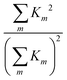of eqn (7) and (10) from ref. 24 where a factor of N appears to be missing. Eqn (7) also appears to be missing a factor of r. To the best of our understanding, to convert the reduced intensity data, si(q), in Fig. 2 of the aforementioned article to our notation as shown in Fig. 2 (top) we must use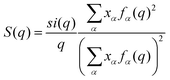. In the case of Fig. 2 (bottom) we use the definition of g*(r) provided in eqn (9) of ref. 24.
 This journal is © the Owner Societies 2020